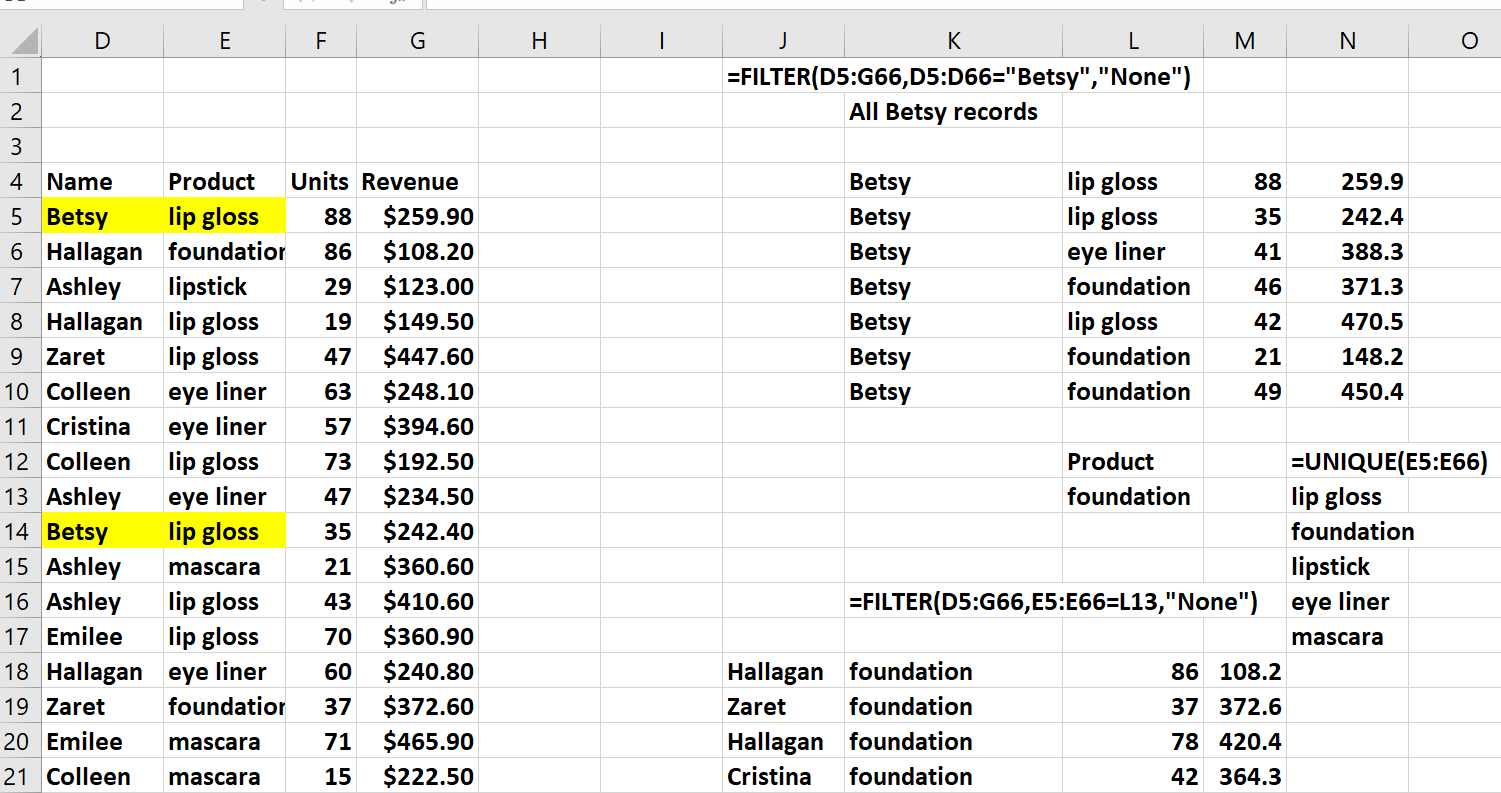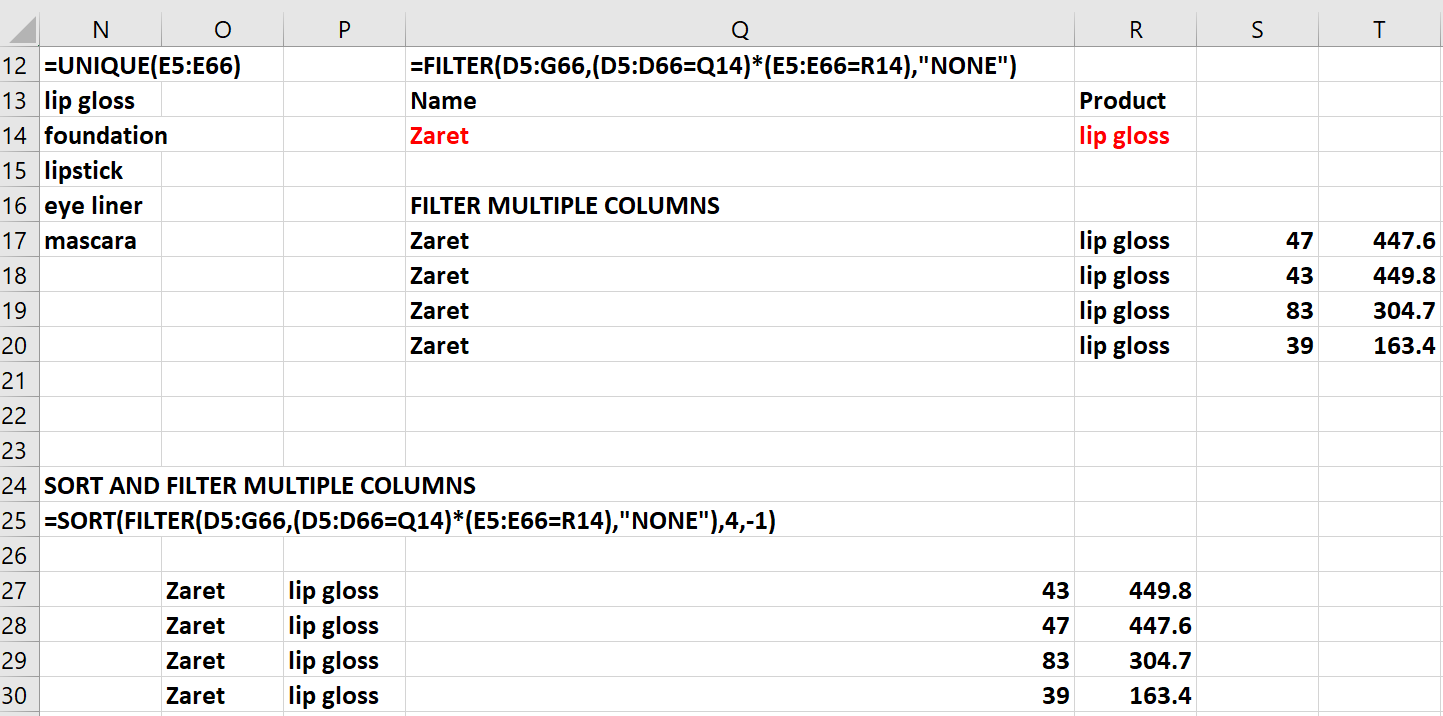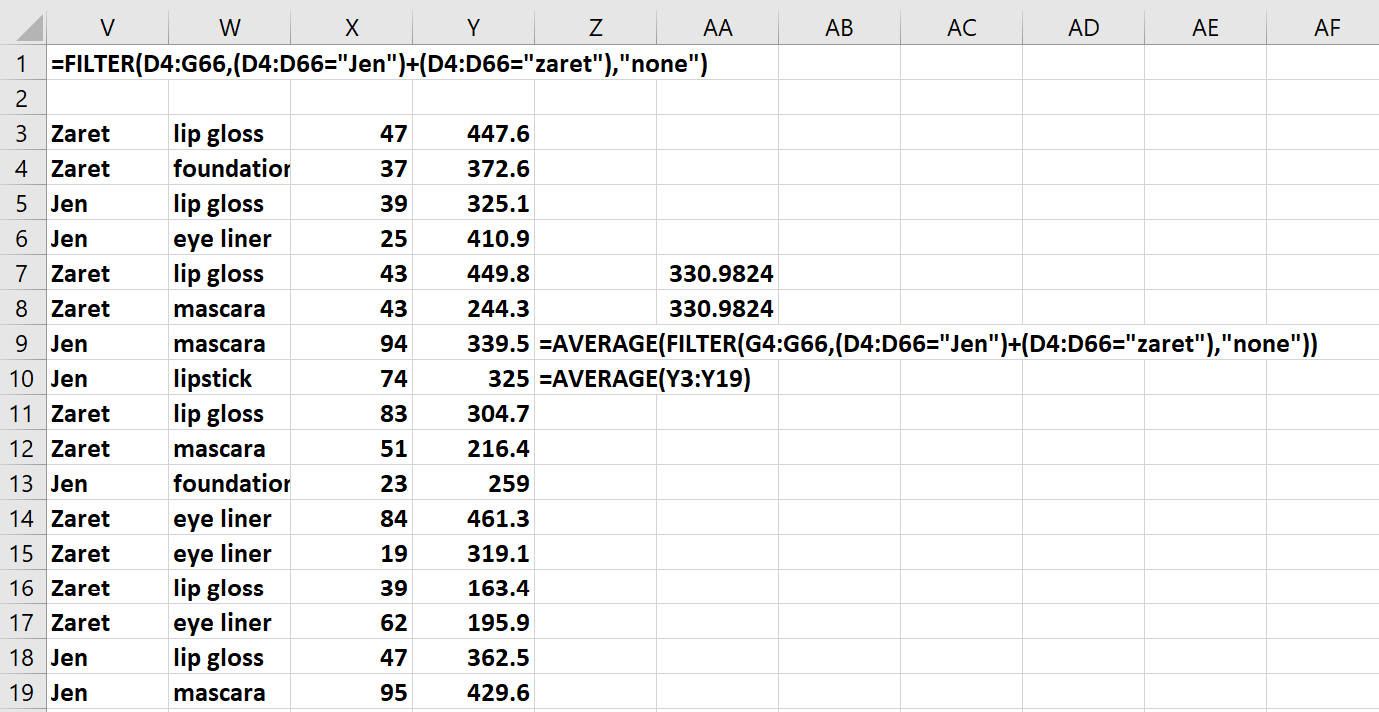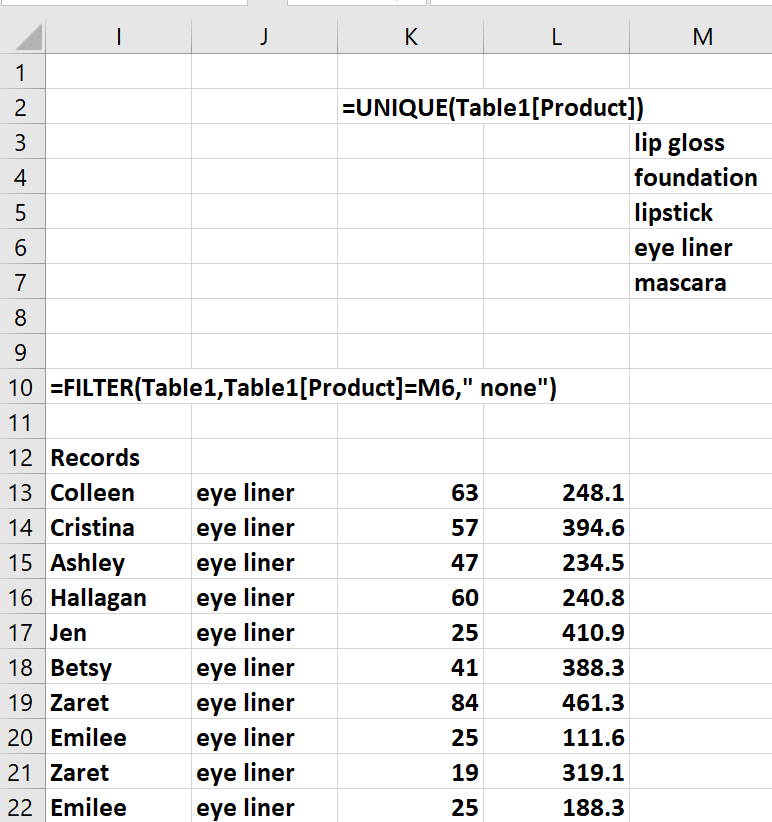# The Amazing FILTER FunctionIf you have Office 365 (recently renamed as Microsoft  365) you now have access to several dynamic array functions that will enable you to perform many time-saving tasks. In our previous posts, we introduced you to the UNIQUE, SORT and SORTBY dynamic array functions. In this article, you will learn how the FILTER function (often combined with other dynamic array functions) can be used to efficiently filter spreadsheet data.

The FILTER function lets you filter data with a function. It is important to note that you can combine the dynamic array functions. In this workbook, we illustrate the use of the FILTER function.

As shown in Figure 1, the cell range D4:G66 of the worksheet “FILTER” contains information on makeup sales transactions.  Each row contains four pieces of information about a transaction:

• Name of salesperson
• Product sold
• Units sold
• Revenue from transaction

Figure 1 - Examples of the FILTER functionFigure 1 also contains two examples of the  FILTER function in action.

• In cell K4, the formula =FILTER(D5:G66,D5:D66="Betsy","None") spits out each row of data in which the Column D entry (Name) is Betsy. If no result is found, then the word None is returned.
• In cell L13, we created a dropdown list of products by entering the formula   =UNIQUE(N14:N18). Then, in cell J18, the formula  =FILTER(D5:G66,E5:E66=L13,"None") returns all rows of data  for which the product sold matches the product selected from cell L13’s dropdown box.

Figure 2 shows two more examples of the FILTER function in action.

Figure 2 - More applications of the Filter function• In cell Q17, the formula  =FILTER(D5:G66,(D5:D66=Q14)*(E5:E66=R14),"NONE") filters our data to show only those rows where Zaret sold lip gloss. The portion (D5:D66=Q14)*(E5:E66=R14) of the formula will be true for a row if and only if the row has Name = Zaret and Product = Lip gloss.
• In cell O27, the formula =SORT(FILTER(D5:G66,(D5:D66=Q14)*(E5:E66=R14),"NONE"),4,-1) returns all rows where Zaret sold lip gloss, and then sorts these rows based on the fourth column of data (revenue) in descending order. The argument -1 makes the sort descending, while the argument +1 (or omitted) makes the sort ascending.  Note that this formula combines two dynamic array functions.

Figure 3 shows how the FILTER function can be used to extract a subset of data using an OR criteria.

Figure 3 - Using the FILTER function with an OR filter• Entering the formula =FILTER(D4:G66,(D4:D66="Jen")+(D4:D66="zaret"),"none") in cell V3 returns each row of data in which the Name is Jen or Zaret. If no such row exists, the word “none” is returned.

In cell AA7, we used the formula =AVERAGE(FILTER(G4:G66,(D4:D66="Jen")+(D4:D66="zaret"),"none")) to average all rows in which Jen or Zaret is the salesperson. Note that this calculation could be completed without extracting the relevant rows.

The Filter Function and Excel Tables

In the worksheet “Table,” we made source data a table by selecting the range D4:G66 and then choosing “Table” from the Insert tab.  The name of the table is Table1 (if desired, you can change the name in the “Table Design” tab that appears on the ribbon when you are in the table.) Any formula referring to Table1 will automatically update when new data is added. As shown in Figure 4, the formula =FILTER(Table1,Table1[Product]=M6," none") returns every row in the table involving eyeliner sales. If you add a new row to the bottom of the table involving eyeliner sales, data for that transaction will automatically be appended to I23:L23.

Figure 4 - Using the FILTER Function with an Excel TableKeep coming back to the Becker blog for more of Dr. Wayne Winston’s best Excel tips!

### Now Leaving Becker.com

You are leaving the Becker.com website. Once you click “continue,” you will be brought to a third-party website. Please be aware, the privacy policy may differ on the third-party website. Adtalem Global Education is not responsible for the security, contents and accuracy of any information provided on the third-party website. Note that the website may still be a third-party website even the format is similar to the Becker.com website.